If you have read Part 1 of this blog post, you should already know how to set up and build a Learning to Rank (LTR) system starting from the available data and using open source libraries.

We ended that post with the part of Future Works where we mentioned three aspects that we would like to address. So this blog post is divided into three main sections:

• In the First Section, we sample the dataset as we are interested in finding out if, having less data, we could still get a good model, hence similar (or better) results than the training with the Full Dataset.
• In the Second Section, we want to understand what happens if we rank the results by directly ordering the values of a specific feature (in our case the Streams descending) and without training any model.
• The Third Section concerns the explanation of the models’ behavior through the use of the library SHAP; in particular, we will see how each feature impacts the output of the models.

Let’s see together, one by one, the results obtained from 3 different implementations that have been performed to solve the above-mentioned problems.

## 1) What would happen if we estimated the Relevance Labels from the song position values, after sampling our dataset?

We sampled our Full Dataset, through the use of a Pandas function that only filters specific rows from the data frame; in particular, we were interested in obtaining a subset containing Daily Song Charts from Position 1 to 21 only:

``subset = data_frame[data_frame["Position"] <= 21]``

We got a Subset of 412.385 observations, around 12% of the Full Dataset, that contains exactly the same data structure (refer to the data preprocessing and feature engineering parts explained in the previous blog post).

We have extracted the training and the test sets (80:20) directly from the training and the test sets of the Full Dataset (this was done for the reason No. 2 in the General Considerations). The feature ‘Position’ (from 1 to 21) was used to estimate the Relevance Rating, our Target variable; therefore the Relevance Labels from 0 to 20 were obtained by reversing the position values in descending order. This is done to make songs in the first position on the chart (Position 1) have the highest Relevance Label (20), and songs in the last position on the chart (Position 21) have the lowest Relevance Label (0).

We then trained the models using the same algorithm and related parameters chosen for the main project (described in Part 1) and we evaluated the performance of the models using the same test sets, in order to compare them on the same “playground”; the results obtained are shown in the table below:### General Considerations:

1. The model training using the Subset was obviously faster
2. We have to be sure not to have intersections between the training and the test sets; it means that the observations of the test sets must be unknown at training time
3. Although they have less data, models built using the Subset have the best performance; one reason could be that the Full Dataset has greater variability than the Subset
4. Applying Doc2Vec encoding on the Track Name variable appears to be better in the Full Dataset rather than the Subset
5. When we used the smaller test set we got better results, although there are no substantial differences. In this domain, the test set’s dimension of the Subset (around 84.000 observations) already gives us sufficient information. Usually, we imagine that the greater the test set, the better it is; actually, there is a threshold beyond which increasing the dimension of the test set does not lead to any advantages.

We also investigated a bit about why the eval ndcg@10 value is greater than the train ndcg@10 when using Doc2Vec encoding (in general it should be the opposite). This is a rare phenomenon but it can happen with any ML model; there are several reasons:

• The test set size may be too small
• The test set consists of “easier” examples than the training set
• There is not so much variance in our dataset
• Our train/test split is suitable for such behavior by accident

Anyway, in this case, the difference is really irrelevant, but if it were to be a very large discrepancy, we need to investigate further. One suggestion is to try using the k-fold cross-validation or, if the model takes too much time to train, just retraining on a differently mixed train/test sets to see if the trend persists.

## 2) In addition, what might change if we directly used Streams counts (descending) to sort the results? Will we get the same order in the search results list (hence maximum NDCG)?!

To find the answer to this question, we used the Full Dataset (with relevance labels from 0 to 20), obtained after the data preprocessing part.

Please note: Relevance Labels are represented by the Ranking column in all the images below

Since our goal was to sort songs by ‘Streams’ count, it is quite useful and interesting to take a look at the Correlation Matrix, a table showing correlation coefficients between variables:We can see that the correlation between Streams and Position (-0.1339) and between Streams and Ranking (0.1697) is very low, while the highest correlation is between Position and Artists (0.4665).

We sorted the Full Dataset in descending order based on the ‘Streams‘ feature, grouping by query_ID, via the following line of code:

``data_frame_sorted = data_frame.groupby(["query_ID"]).apply(lambda x: x.sort_values(["Streams"], ascending=False)).reset_index(drop=True)``

Once we obtained the ordered dataset, we manually calculated the NDCG@10 through a part of code where we directly apply the DCG formula and its normalized variant only on the top 10 songs of the search result list for each query. Below you can see an example of the first 10 results returned for both query_ID = 0 and query_ID = 1, respectively.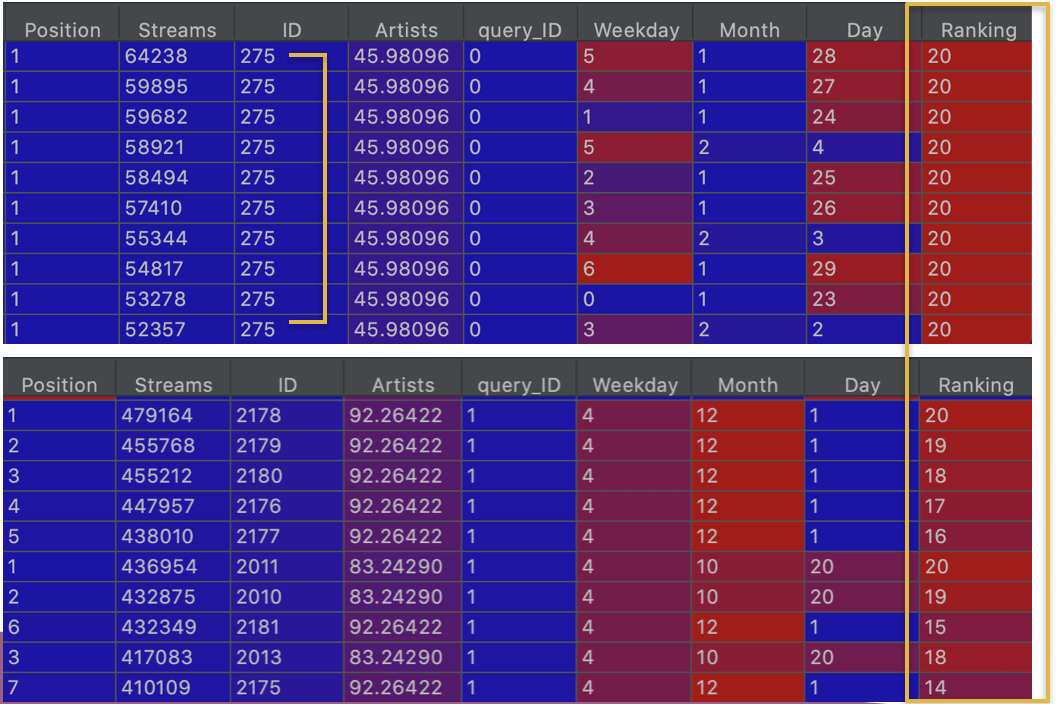On the sorted dataset, we applied the following steps to the ‘Ranking’ column (for each query):

• Calculate the DCG@10 

• Calculate the Ideal DCG@10

• Calculate the NDCG@10 = DCG@10/ Ideal DCG@10

• Average the NDCG@10 values across all the queries

The final result was NDCG@10 = 0.9347

Without training any models, we got good (and better) NDCG by directly using the Streams counts in descending order to sort the songs. As you can see from the picture above, for query 0 the maximum streams are all related to the songs in the top position on the chart (Ranking 20), while for query 1 the maximum streams are related to the higher positions on the chart but the results are scattered.

Furthermore, we also noticed that for some queries (as query 0) the first results of the ordered list were always represented by the same song (identical ID), on different days. Hypothetically a search engine that returns a list of identical items (same songs) does not make sense. We have decided to keep only the observation with the maximum number of streams for each song and for each query: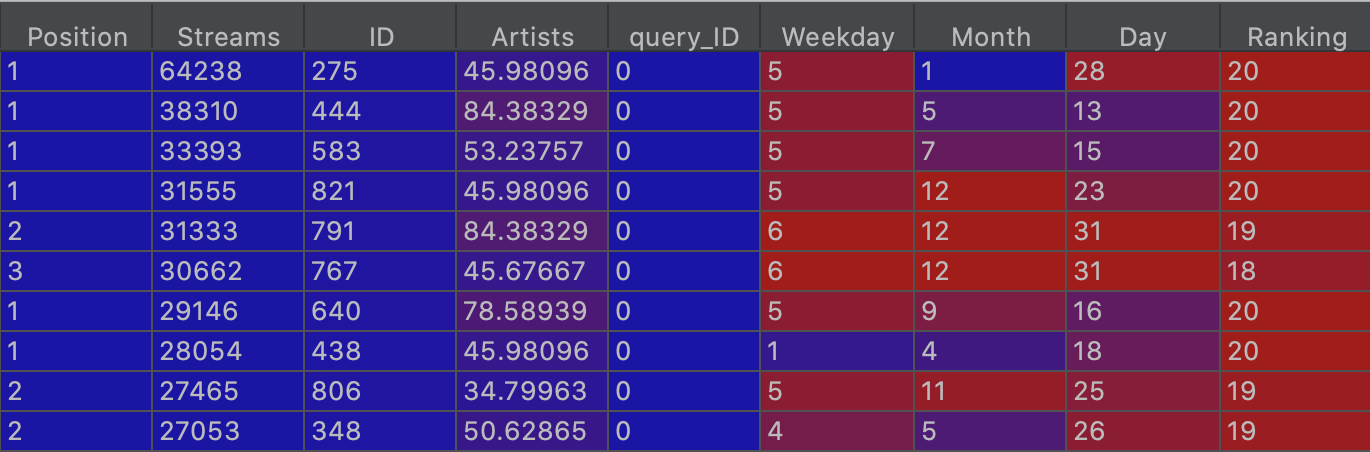After sorting again in descending order (as you can see from the image above about query 0), the final result (thus the average across all the queries) was NDCG@10 = 0.8212. In this case, removing some observations got the performance worse.

#### Subset

We repeated the same experiment using the Subset rather than the Full Dataset and these were the observed results:

1. Less Correlation between variables:
• Correlation between Position and Streams is:  -0.0810
• Correlation between Ranking and Streams is:  0.0810
• Correlation between Position and Artists is: 0.1563
2. Same NDCG@10 (0.9347)
3. NDCG@10 slightly lower (0.8176 instead of 0.8212), when we have kept only the observation with the maximum streams for each song.

## 3) Give an explanation of the model behavior through the use of the library SHAP, in particular how each feature impacts the model’s output

If you missed our previous blog post about SHAP, read it first to learn about the amazing tools of this library and how to explain Learning to Rank Models using the TreeSHAP algorithm.

As illustrated so far, we have created a dataset (Full) and a Subset, we have trained several types of models using different data preprocessing techniques, and now we would like to understand how these models achieve those results.

We have made a comparison between 4 different models:SHAP library  creates explanations of the model wondering for every prediction and feature how the prediction x change if feature y is removed from the model. The so-called SHAP values are the answer.

Since we built LTR models using LambdaMART (Multiple Additive Regression Trees), we used the TreeExplainer , an algorithm to compute SHAP values for trees and ensembles of trees, in polynomial time.

TreeSHAP provides us with several different types of plots, each one highlighting a specific aspect of the model. Matplotlib, a highly useful visualization library, is used for the rendering of the graphs in Python.

#### SUMMARY PLOT

The summary plot gives us Global Interpretability. The shap.summary_plot function with plot_type = “bar”, let you produce the feature importance plot (variables ranked in descending order) with the mean(|SHAP value|) in the x-axis.We can see that the Streams feature is actually the most important, followed by the Artists feature.

If you want to show the positive and negative relationship of the predictors with the target variable, you have to produce the other type of summary plots:These plots show not only variable importance (from top to bottom) but also shows how the feature values (using the color spectrum from blue to red) affect the label prediction; each observation (song), has a dot on each row.

Here, the higher the number of Streams the higher the positive impact on the relevance (SHAP value above zero); whereas the ‘Artists’ feature is negatively correlated with the target variable. If you remember, the Artists column was encoded using the Leave One Out technique, which replaces the categorical values with the mean of the target variable values related to that level but excludes the current row’s target value when calculating the mean; therefore this numerical value is not significant in itself but it would be more interesting to know which Artist corresponds to that value to understand who is the most popular.

The ranking of the other features looks a bit different but overall we can say that the Subset is sufficiently representative of the Full dataset.

#### DECISION PLOT

The decision plot shows how models make decisions, displaying the cumulative effect of each feature. Each vertical line represents a single prediction: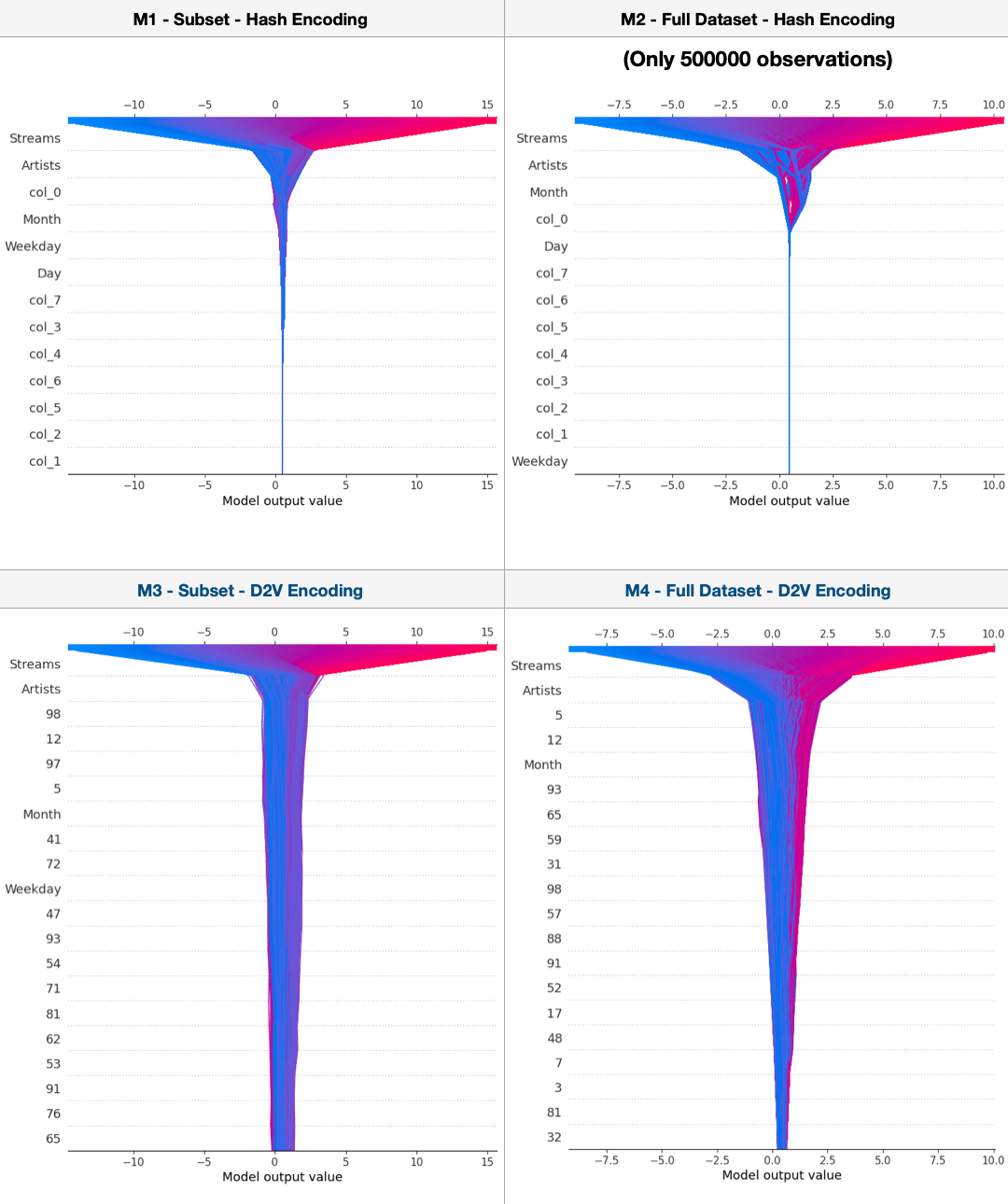The system was unable to get this graph with the Full dataset so we only got it for 500.000 observations. The interesting aspect is that in the M1 and M2, the features that have been encoded (col_0 to col_7) interfere slightly and the lines are almost straight (maybe because they are binary variables); on the other hand, in M3 and M4 there is more variability but the encoded features (0 to 99) are too much and it is not easy to tell which Track Name has the most impact.

To better understand the decision plot, let’s consider only one observation. The following graphs display the effects of the different features’ values on the single selected prediction, and if they impact positively or negatively on the model. In our case we always plotted the same observation (song) in different models: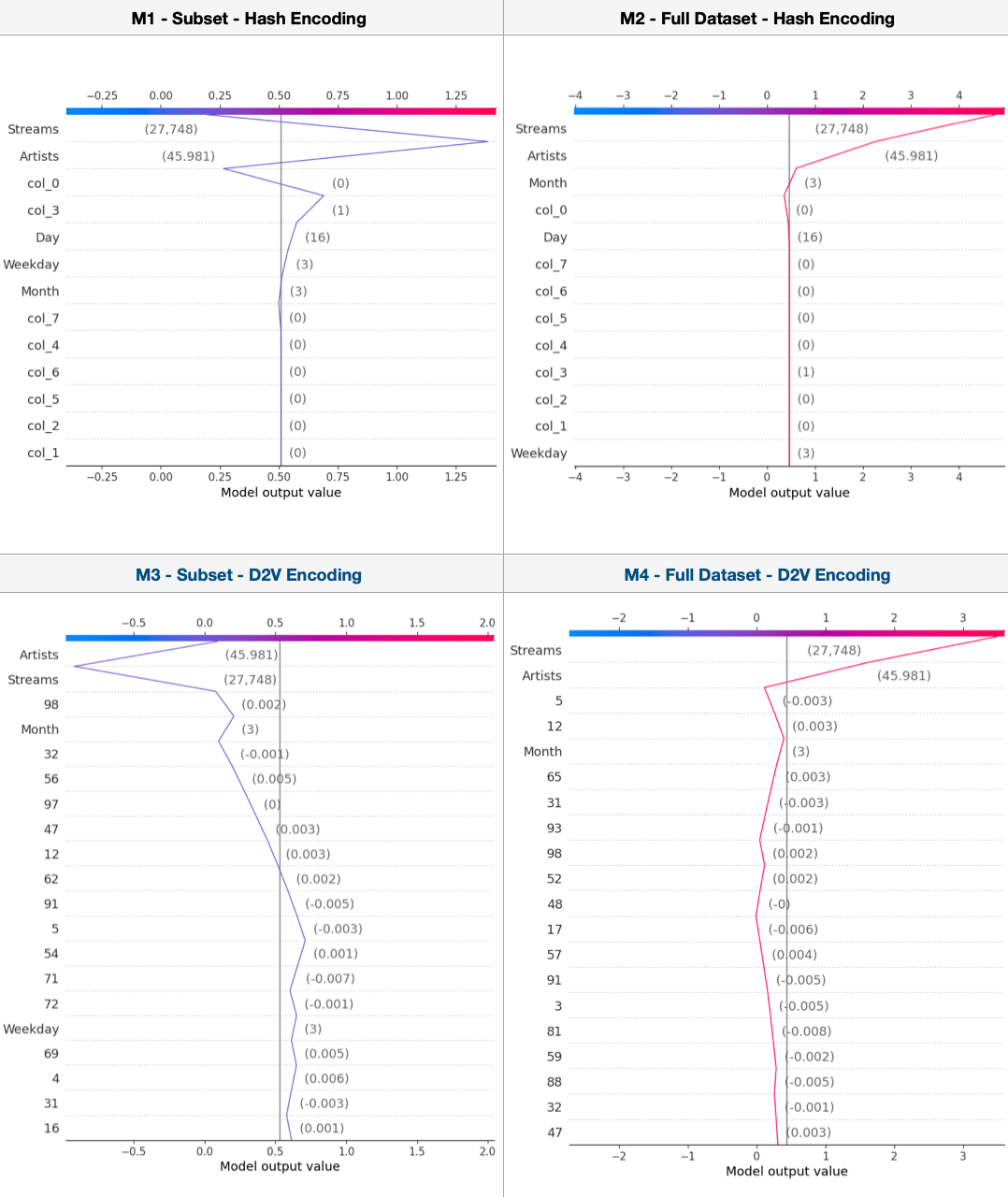For example, in the first graph, we can see that Month 3, Weekday 3, Day 16, col_3 True, Artists 45.981 impact positively to the model, while the other features impact negatively.

It is interesting to say that the same feature value has different impacts on the final model output; moreover using the Full Dataset we obtained model’s output values greater than those when we used the Subset (4.79 in M2 against 0.19 in M1 and 3.52 in M4 against 0.09 in M3). The difference is substantial: the reason could be that removing some observations worsens the importance of that same observation.

#### FORCE PLOT

This plot gives us a Local Interpretability, showing the SHAP value for a single observation. For simplicity, let’s take the same observation used above and show only one Force graph, representing M1: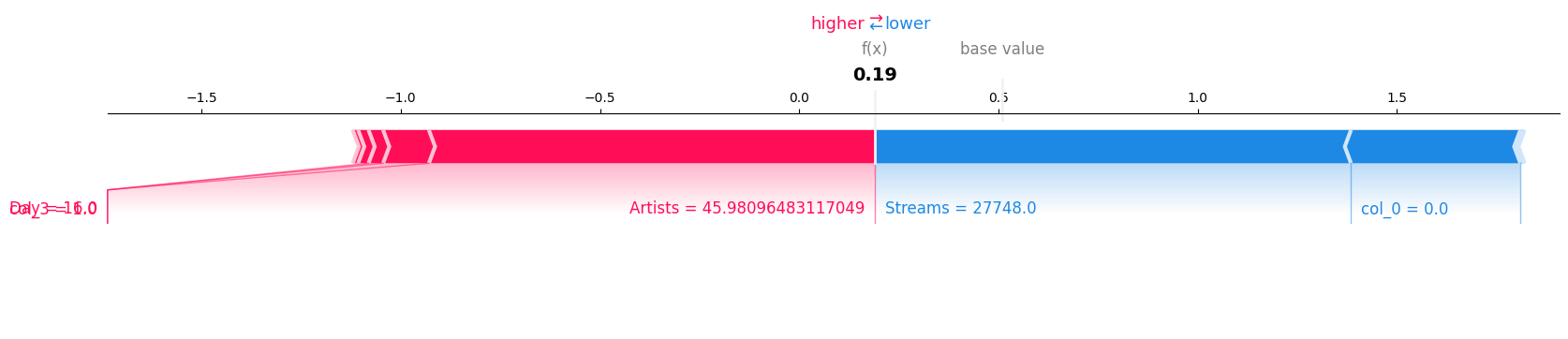From this plot, you can see:

• f(x) is the model prediction (0.19)
• the base value, that is the value that would be predicted if we did not know any features for the current output
• how and how much each feature impacts the output: features pushing the prediction higher are shown in red and those pushing the prediction lower are in blue. The fact that the ‘Artists’ is 45.9809 (that is Luis Fonsi) impacts positively, while the fact that the ‘Streams’ is 27748 impacts negatively.

Now supposed to consider 4 different observations and to check their model’s output values. The SHAP scores of these songs in answer to a specific query (for example query_ID = 0 that corresponds to Ecuador Region) are reported and sorted in the following table:The output of the model is not the relevance label, but it represents the same concept if we look at the relative relevance of the SHAP scores between songs. Since 0.19 > -4.42 > -4.96 > -9.02, the ranking of the results will be equivalent both using the SHAP scores or the relevance labels.

#### DEPENDENCE PLOT

The dependence plot shows the marginal effect that two features have on the predicted outcome of a model. As a first example, I reported the dependence plots between Streams and Artists:Here each point corresponds to a prediction; on the x-axis, we have the Streams values,  on the y-axis, we have the predicted SHAP values and the colors represent the Artists. We can see a very strange situation: when the number of Streams is very low, each Artist really makes the difference; vice versa after a certain threshold each artist impacts in the same way, and the SHAP values are high and constant.

As a second example, I reported the dependence plots using the same features but inverting them on the axes: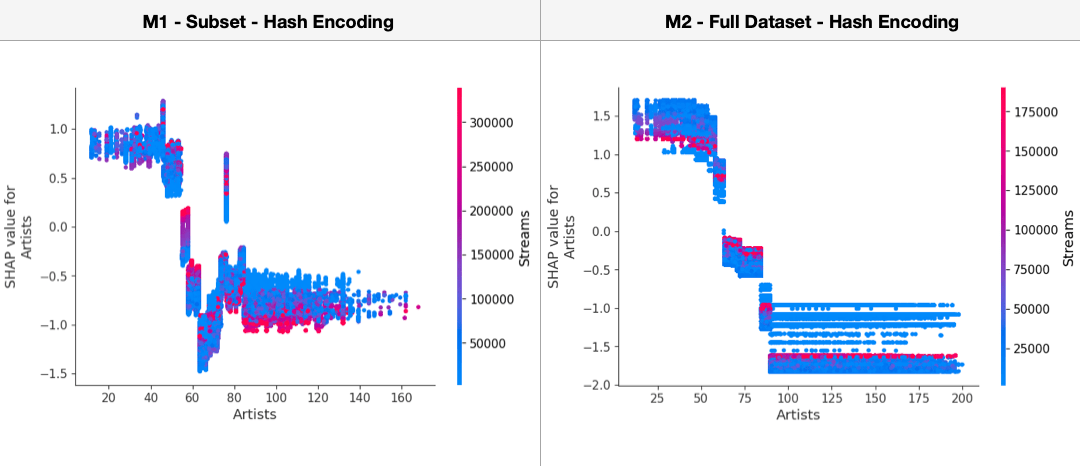Even in this case, we can notice that ‘Artists’ make the difference: Artists’ values < 50 (plus or minus) produce higher SHAP values than the others. As mentioned above, the ‘Artists’ feature has been encoded with a technique based on the mean of the target variable values (Position on chart) so the Artists who are known to always be in the highest positions are also those who have the lowest encoding values (so the average of the Position values < 50). This result suggests that Artists who have the same relevance, also have the same impact on the model; therefore the most popular Artists positively affect the model’s output.

We can also create this kind of plot for the date of the charts, that is, for day, month and weekday features respectively and we colored the dots according to the number of Streams:In this case, we can immediately see that day and weekday have no impact on the model output in the Full Dataset (this reflects the results of the summary plot). There are only some days with more streams than others (Sunday for example).

In the Subset, regardless of the Streams, we can notice that the first half of the month, the first half of the year, and the first half of the week produce greater SHAP values than the second half, which means a positive impact on the output. Anyway, without additional information, it is difficult to explain this type of behavior.

### Summary

Let’s recap the main points of the Learning to Rank project on a Daily Song Ranking problem.

In the first blog post, we illustrated the pipeline to set up and build a Learning to Rank (LTR) system starting from the available data, creating and manipulating the training set and the test set and then training a ranking model using open source libraries. In the second blog post, we performed further analyses by playing with the data to find insights and provided an explanation of the models’ behavior through the use of the library SHAP. All the obtained results were computed on the Spotify dataset about Worldwide Daily Song Ranking available on Kaggle.

### TAKEAWAYS

• Data Preprocessing and Feature Engineering are crucial and are the parts we should pay more attention to
• Doc2vec encoding technique seems to be better than the Hash encoding in model performance when we have a greater dataset
• A smaller dataset may have less variability and may be easier to understand but less representative
• It may happen that the eval ndcg@10 value is greater than the train ndcg@10 value
• Especially in some domains, there is a threshold beyond which increasing the size of the test set does not lead to any improvement
• Without training any model, we could get a good ranking of the results by directly ordering (descending or ascending) the values of a specific feature
• The SHAP library is a powerful tool for explaining the importance of the feature. Several graphs show us many insights that help in understanding how each variable impacts the output of the models; however, without a good knowledge of the context in which we are working, it is not always easy to give exhaustive explanations.

### Future Works

Until now, we have only generated the ‘query_ID‘ from the Region column, without considering the date of the song charts. During the analysis described in the second section of this blog post, we realized that we could consider it as a hash of multiple query-level features, in order to avoid “duplicates” of songs for each query. In the next blog post, we will create the query_ID as a hash of multiple variables (Region, Day, Month, and Weekday) and we will make a comparison between the obtained models again.

I hope these two blog posts have been interesting and that you have learned the tools needed to tackle an LTR task and carry out related investigations.

##### References

 SHAP: https://github.com/slundberg/shap

// STAY ALWAYS UP TO DATE